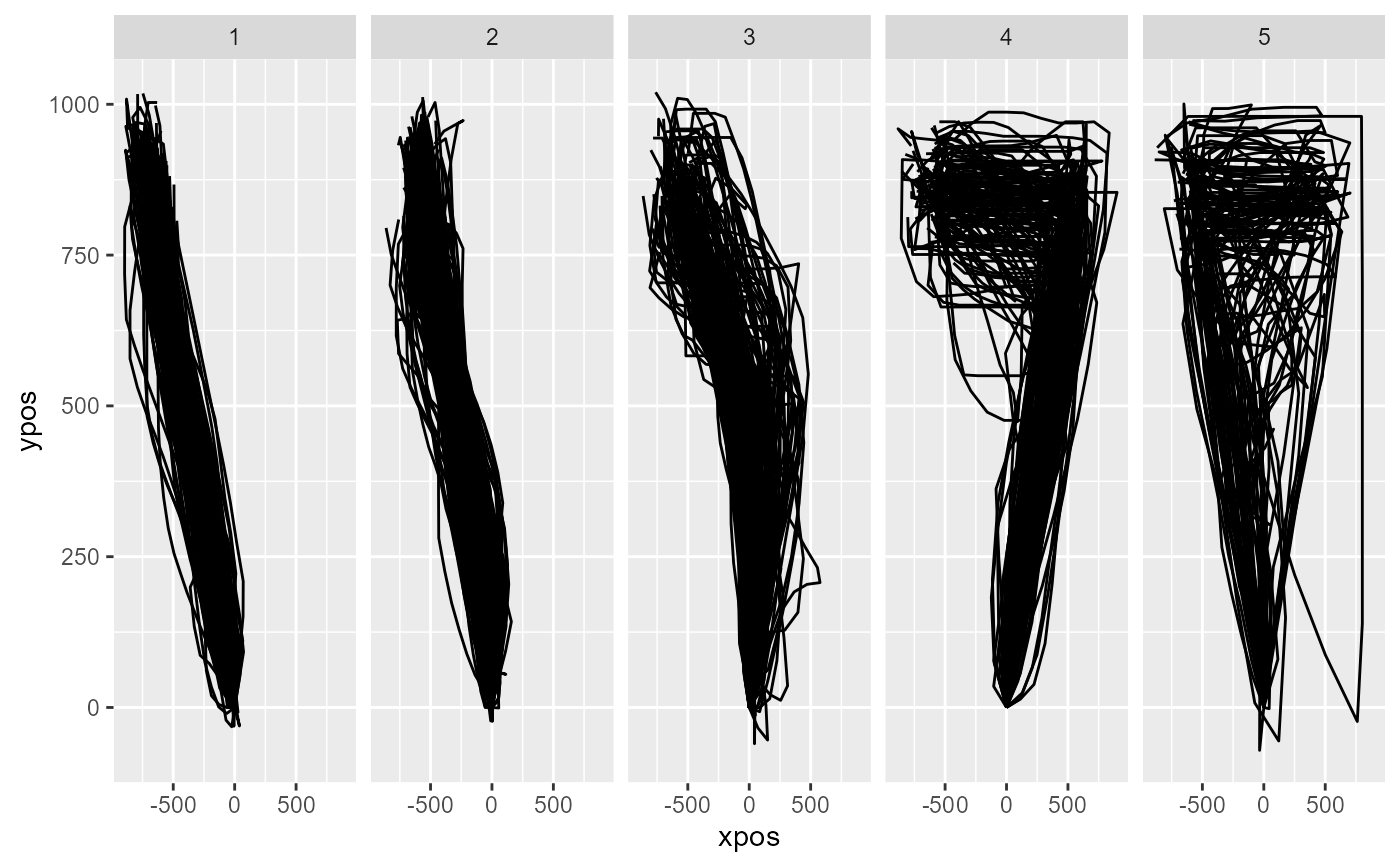Performs trajectory clustering. It first computes distances between each pair of trajectories and then applies off-the-shelf clustering tools to explain the resulting dissimilarity matrix using a predefined number of clusters.

mt_cluster(
data,
use = "ln_trajectories",
save_as = "clustering",
dimensions = c("xpos", "ypos"),
n_cluster = 5,
method = "hclust",
weights = rep(1, length(dimensions)),
pointwise = TRUE,
minkowski_p = 2,
hclust_method = "ward.D",
kmeans_nstart = 10,
na_rm = FALSE,
cluster_output = FALSE,
verbose = FALSE
)

## Arguments

data

a mousetrap data object created using one of the mt_import functions (see mt_example for details). Alternatively, a trajectory array can be provided directly (in this case use will be ignored).

use

a character string specifying which trajectory data should be used.

save_as

a character string specifying where the resulting data should be stored.

dimensions

a character vector specifying which trajectory variables should be used. Can be of length 2 or 3, for two-dimensional or three-dimensional trajectories respectively.

n_cluster

an integer specifying the number of clusters to estimate.

method

character string specifying the clustering procedure. Either hclust (the default) or kmeans.

weights

numeric vector specifying the relative importance of the variables specified in dimensions. Defaults to a vector of 1s implying equal importance. Technically, each variable is rescaled so that the standard deviation matches the corresponding value in weights. To use the original variables, set weights = NULL.

pointwise

boolean specifying the way in which dissimilarity between the trajectories is measured. If TRUE (the default), mt_distmat measures the average dissimilarity and then sums the results. If FALSE, mt_distmat measures dissimilarity once (by treating the various points as independent dimensions). This is only relevant if method is "hclust". See mt_distmat for further details.

minkowski_p

an integer specifying the distance metric for the cluster solution. minkowski_p = 1 computes the city-block distance, minkowski_p = 2 (the default) computes the Euclidian distance, minkowski_p = 3 the cubic distance, etc. Only relevant if method is "hclust". See mt_distmat for further details.

hclust_method

character string specifying the linkage criterion used. Passed on to the method argument of hclust. Default is set to ward.D. Only relevant if method is "hclust".

kmeans_nstart

integer specifying the number of reruns of the kmeans procedure. Larger numbers minimize the risk of finding local minima. Passed on to the nstart argument of kmeans. Only relevant if method is "kmeans".

na_rm

logical specifying whether trajectory points containing NAs should be removed. Removal is done column-wise. That is, if any trajectory has a missing value at, e.g., the 10th recorded position, the 10th position is removed for all trajectories. This is necessary to compute distance between trajectories.

cluster_output

logical. If FALSE (the default), the mousetrap data object with the cluster assignments is returned (see Value). If TRUE, the output of the cluster method (kmeans or hclust) is returned directly.

verbose

logical indicating whether function should report its progress.

## Value

A mousetrap data object (see mt_example) with an additional data.frame added to it (by default called clustering) that contains the cluster assignments. If a trajectory array was provided directly as data, only the clustering data.frame will be returned.

## Details

mt_cluster uses off-the-shelf clustering tools, i.e., hclust and kmeans, for cluster estimation. Cluster estimation using hclust relies on distances computed by mt_distmat.

Mouse trajectories often occur in distinct, qualitative types (see Wulff et al., 2019; Wulff et al., 2022). Common trajectory types are linear trajectories, mildly and strongly curved trajctories, and single and multiple change-of-mind trials (see also mt_map). mt_cluster can tease these types apart.

mt_cluster uses hclust or kmeans to explain the distances between every pair of trajectories using a predefined number of clusters. If method is "hclust", mt_cluster computes the dissimiliarity matrix for all trajectory pairs using mt_distmat. If method is "kmeans", this is done internally by kmeans.

We recommend setting method to hclust using ward.D as the linkage criterion (via hclust_method). Relative to kmeans, the other implemented clustering method, and other linkage criteria, this setup handles the skewed distribution cluster sizes and trajectory outliers found in the majority of datasets best.

For clustering trajectories, it is often useful that the endpoints of all trajectories share the same direction, e.g., that all trajectories end in the top-left corner of the coordinate system (mt_remap_symmetric or mt_align can be used to achieve this). Furthermore, it is recommended to use length normalized trajectories (see mt_length_normalize; Wulff et al., 2019, Wulff et al., 2023).

mt_distmat for more information about how the distance matrix is computed when the hclust method is used.

mt_cluster_k for estimating the optimal number of clusters.

## Author

Dirk U. Wulff

Jonas M. B. Haslbeck

## Examples

# Length normalize trajectories
KH2017 <- mt_length_normalize(KH2017)

# Cluster trajectories
KH2017 <- mt_cluster(KH2017, use="ln_trajectories")

# Plot clustered trajectories
mt_plot(KH2017,use="ln_trajectories",
use2="clustering",facet_col="cluster")